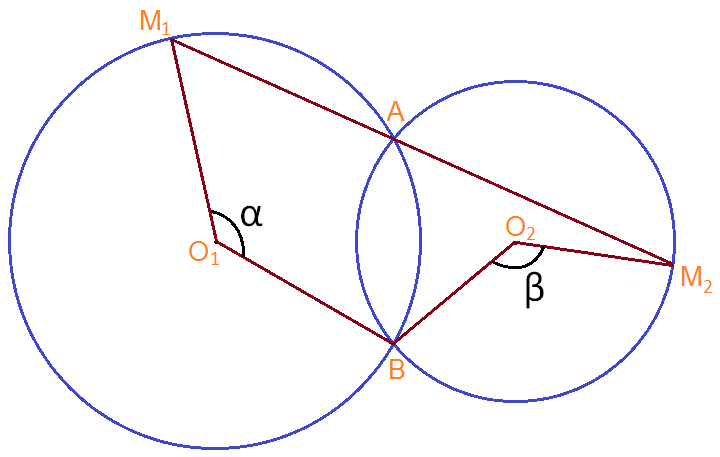# Cool geometry problem - 4

Geometry Level 1Two circles centered at $O_{1}$ and $O_{2}$ intersect at points $A$ and $B$. A line is drawn through point $A$; the line intersects the first circle at point $M_{1}$ and the second circle at point $M_{2}$.

Which of the following is true ?

×